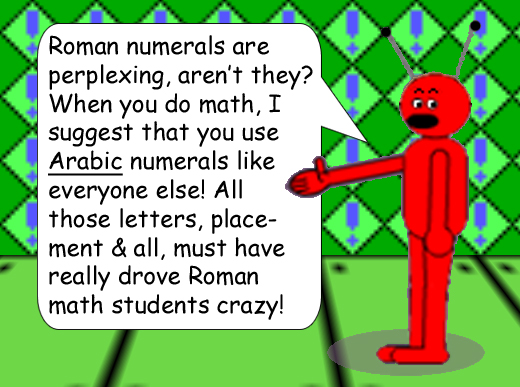# Roman Numeral Twist

## And this is the Roman numeral for 1,100: MC

Did you notice how the letters switched places for each 2nd numeral? I call this the Roman Numeral Twist!## II * X^p

(I replaced the "x" multiplication symbol with an asterisk & the exponent variable "x" with a lowercase P to prevent confusion with the "X" Roman numeral for number 10.) The exponent must be an integer!

Do you understand the logic with the Roman numerals at the top of this Web page? The numbers 4, 6, 9 & 11 × 10 to the power of an integer of your choice is printed using this logic:

Subtract if the smaller numeral is at the left of the bigger one; add if the smaller numeral is at the right of the bigger one.

## CM + CC = MC & MC - CC = CM

What about Roman numerals for numbers greater than or equal to 4,000? There's another Web page on the Math Trick Menu with more information. See "Why Numbers Are Important".

## MMMCMXCIX